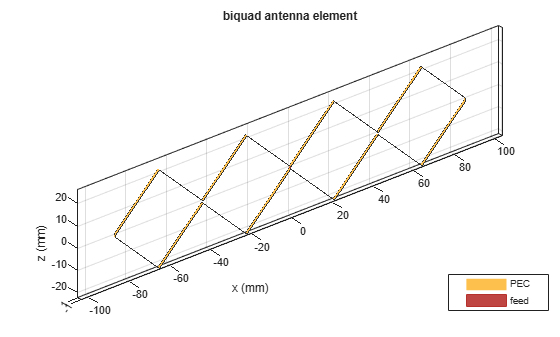## Description

The `biquad` antenna is center fed and symmetric about its origin. The default length is chosen for an operating frequency of 2.8 GHz.

The width of the strip is related to the diameter an equivalent cylinder:

`$w=2d=4r$`

, where:

• d is the diameter of equivalent cylindrical dipole.

• r is the radius of equivalent cylindrical dipole.

For a given cylinder radius, use the `cylinder2strip` utility function to calculate the equivalent width. The default strip dipole is center-fed. The feed point coincides with the origin. The origin is located on the yz- plane.## Creation

### Syntax

``bq = biquad``
``bq = biquad(Name,Value)``

### Description

````bq = biquad` creates a biquad antenna.```

example

``` `bq = biquad(Name,Value)` creates a biquad antenna with additional properties specified by one or more name-value pair arguments. `Name` is the property name and `Value` is the corresponding value. You can specify several name-value pair arguments in any order as `Name1`, `Value1`, `...`, `NameN`, `ValueN`. Properties not specified retain their default values.```

## Properties

expand all

Number of loops for the biquad, specified as a scalar integer. Setting this property to 4 supports a double biquad antenna.

Example: `'NumLoops'`,`4`

Data Types: `double`

Length of two arms, specified as a scalar in meters. The default length is chosen for an operating frequency of 2.8 GHz.

Example: `'ArmLength'`,`0.0206`

Data Types: `double`

Biquad arm width, specified as a scalar in meters.

Example: `'Width'`,`0.006`

Data Types: `double`

Angle formed by biquad arms to the xy- plane, specified a scalar in meters.

Example: `'ArmElevation'`, `50`

Data Types: `double`

Type of the metal used as a conductor, specified as a metal material object. You can choose any metal from the `MetalCatalog` or specify a metal of your choice. For more information, see `metal`. For more information on metal conductor meshing, see Meshing.

Example: ```m = metal('Copper'); 'Conductor',m```

Example: ```m = metal('Copper'); ant.Conductor = m```

Lumped elements added to the antenna feed, specified a lumped element object. For more information, see `lumpedElement`.

Example: `'Load'`, lumpedelement. `lumpedelement` is the object for the load created using `lumpedElement`.

Example: ```bq.Load = lumpedElement('Impedance',75)```

Tilt angle of the antenna in degrees, specified as a scalar or vector. For more information, see Rotate Antennas and Arrays.

Example: `90`

Example: `Tilt=[90 90]`,`TiltAxis=[0 1 0;0 1 1]` tilts the antenna at 90 degrees about the two axes defined by the vectors.

Data Types: `double`

Tilt axis of the antenna, specified as one of these values:

• Three-element vector of Cartesian coordinates in meters. In this case, each coordinate in the vector starts at the origin and lies along the specified points on the x-, y-, and z-axes.

• Two points in space, specified as a 2-by-3 matrix corresponding to two three-element vectors of Cartesian coordinates. In this case, the antenna rotates around the line joining the two points.

• `"x"`, `"y"`, or `"z"` to describe a rotation about the x-, y-, or z-axis, respectively.

Example: `[0 1 0]`

Example: `[0 0 0;0 1 0]`

Example: `"Z"`

Data Types: `double` | `string`

## Object Functions

 `show` Display antenna, array structures, shapes, or platform `info` Display information about antenna or array `axialRatio` Axial ratio of antenna `beamwidth` Beamwidth of antenna `charge` Charge distribution on antenna or array surface `current` Current distribution on antenna or array surface `design` Design prototype antenna or arrays for resonance around specified frequency or create AI-based antenna from antenna catalog objects `efficiency` Radiation efficiency of antenna `EHfields` Electric and magnetic fields of antennas or embedded electric and magnetic fields of antenna element in arrays `impedance` Input impedance of antenna or scan impedance of array `mesh` Mesh properties of metal, dielectric antenna, or array structure `meshconfig` Change meshing mode of antenna, array, custom antenna, custom array, or custom geometry `optimize` Optimize antenna or array using SADEA optimizer `pattern` Plot radiation pattern and phase of antenna or array or embedded pattern of antenna element in array `patternAzimuth` Azimuth plane radiation pattern of antenna or array `patternElevation` Elevation plane radiation pattern of antenna or array `rcs` Calculate and plot radar cross section (RCS) of platform, antenna, or array `returnLoss` Return loss of antenna or scan return loss of array `sparameters` Calculate S-parameters for antennas and antenna arrays `vswr` Voltage standing wave ratio (VSWR) of antenna or array element

## Examples

collapse all

Create a biquad antenna with arm angles at 50 degrees and view it.

```bq = biquad('ArmElevation',50); show(bq)```Calculate the impedance of a biquad antenna over a frequency span 2.5GHz-3GHz.

```bq = biquad('ArmElevation',50); impedance(bq,linspace(2.5e9,3e9,51));```Create and view a double biquad antenna using default property values.

`ant = biquad('NumLoops',4)`
```ant = biquad with properties: NumLoops: 4 ArmLength: 0.0305 ArmElevation: 45 Width: 1.0000e-03 Conductor: [1x1 metal] Tilt: 0 TiltAxis: [1 0 0] Load: [1x1 lumpedElement] ```
`show(ant)`## Version History

Introduced in R2015b